# 2d density plot with ggplot2

This post introduces the concept of 2d density chart and explains how to build it with R and ggplot2. 2d histograms, hexbin charts, 2d distributions and others are considered.

# The issue with `geom_point()`

A 2d density plot is useful to study the relationship between 2 numeric variables if you have a huge number of points.

To avoid overlapping (as in the scatterplot beside), it divides the plot area in a multitude of small fragment and represents the number of points in this fragment.

There are several types of 2d density plots. Each has its proper ggplot2 function. This post describes all of them.``````# Library
library(tidyverse)

# Data
a <- data.frame( x=rnorm(20000, 10, 1.9), y=rnorm(20000, 10, 1.2) )
b <- data.frame( x=rnorm(20000, 14.5, 1.9), y=rnorm(20000, 14.5, 1.9) )
c <- data.frame( x=rnorm(20000, 9.5, 1.9), y=rnorm(20000, 15.5, 1.9) )
data <- rbind(a,b,c)

# Basic scatterplot
ggplot(data, aes(x=x, y=y) ) +
geom_point()``````

# 2d Histogram with `geom_bin2d()`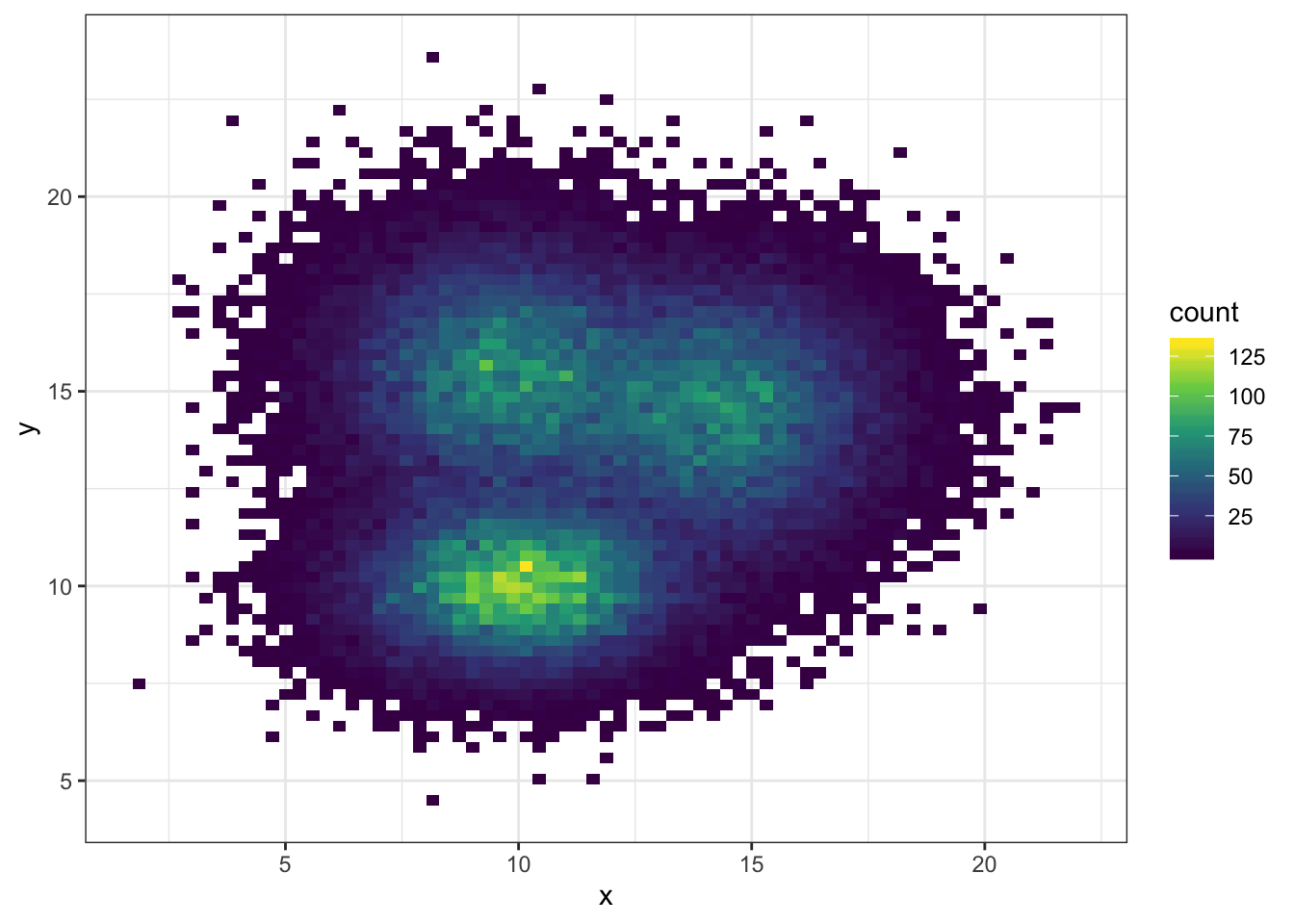For 2d histogram, the plot area is divided in a multitude of squares. (It is a 2d version of the classic histogram). It is called using the `geom_bin_2d()` function. This function offers a `bins` argument that controls the number of bins you want to display.

Note: If you’re not convinced about the importance of the `bins` option, read this.

``````# 2d histogram with default option
ggplot(data, aes(x=x, y=y) ) +
geom_bin2d() +
theme_bw()

# Bin size control + color palette
ggplot(data, aes(x=x, y=y) ) +
geom_bin2d(bins = 70) +
scale_fill_continuous(type = "viridis") +
theme_bw()``````

# Hexbin chart with `geom_hex()`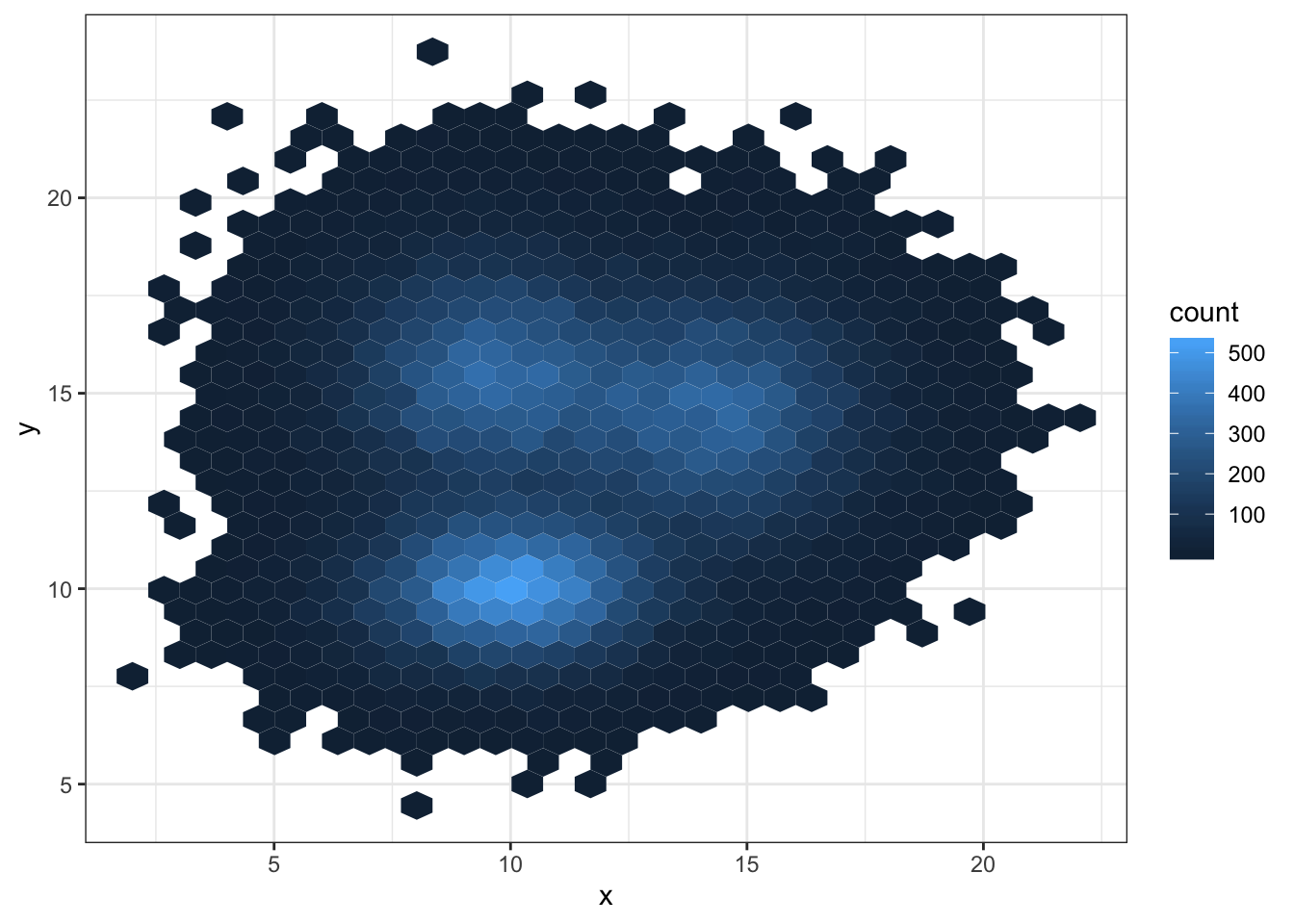Another alternative is to divide the plot area in a multitude of hexagons: it is thus called a hexbin chart, and is made using the `geom_hex()` function.

This function provides the `bins` argument as well, to control the number of division per axis.

``````# Hexbin chart with default option
ggplot(data, aes(x=x, y=y) ) +
geom_hex() +
theme_bw()

# Bin size control + color palette
ggplot(data, aes(x=x, y=y) ) +
geom_hex(bins = 70) +
scale_fill_continuous(type = "viridis") +
theme_bw()``````

# 2d distribution with `geom_density_2d` or `stat_density_2d`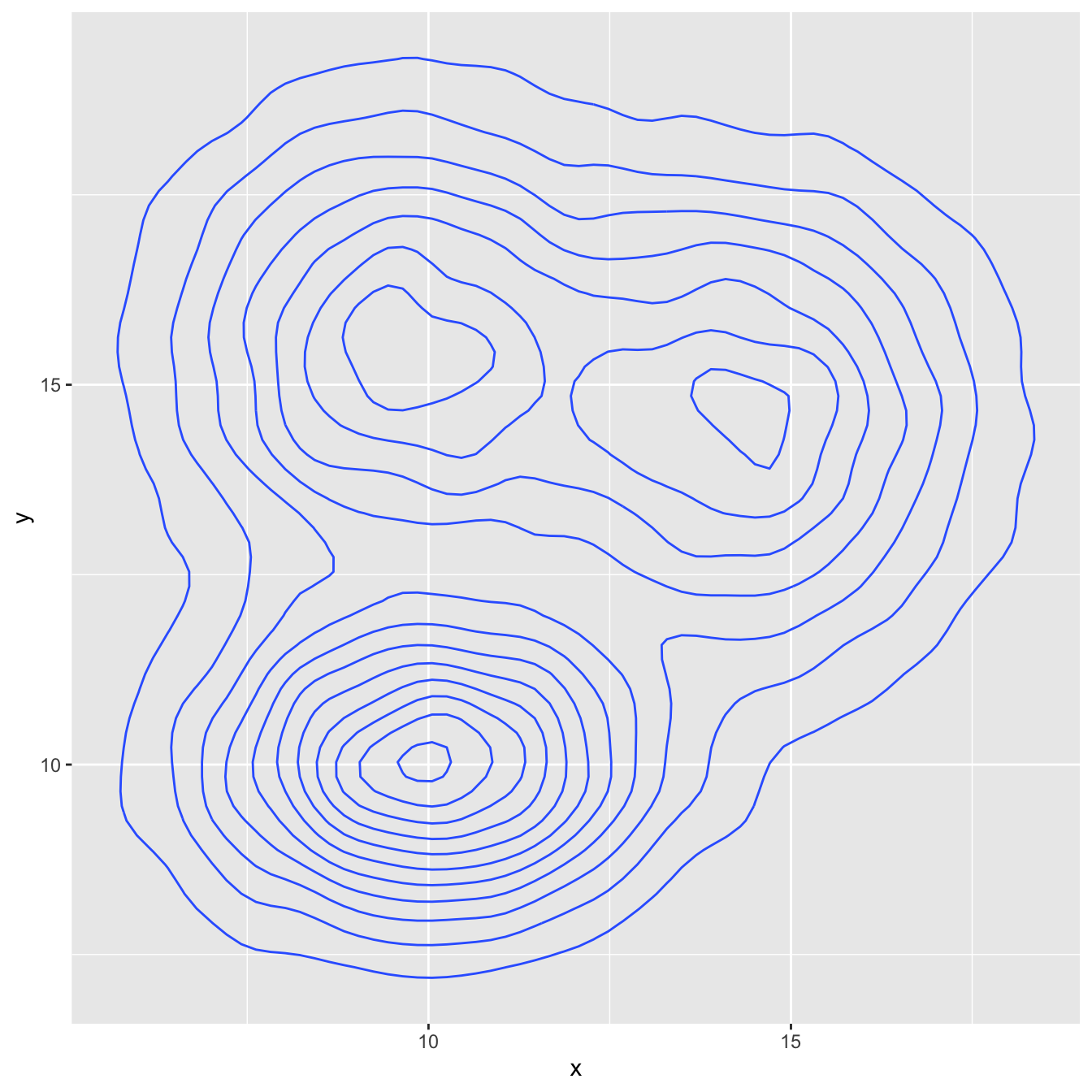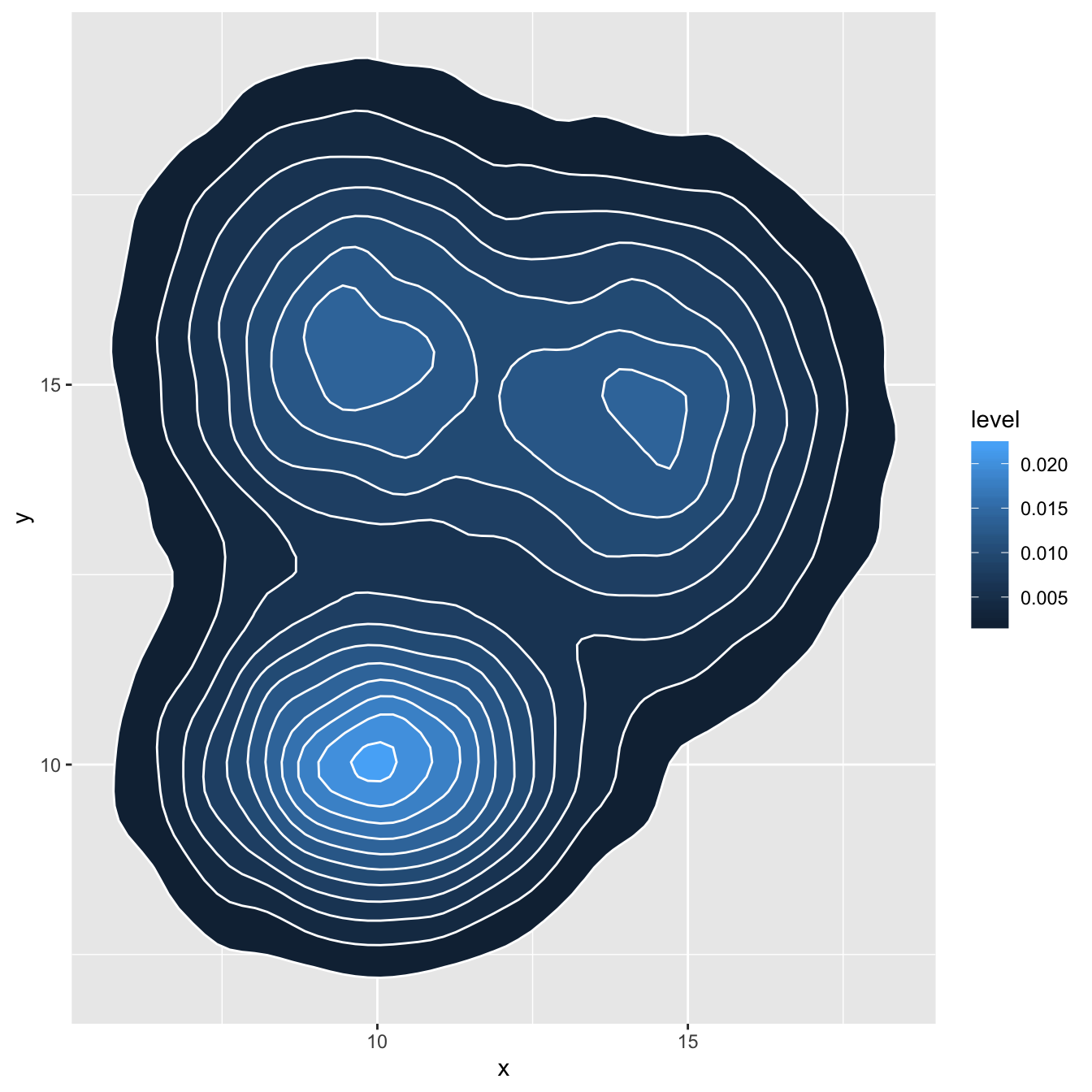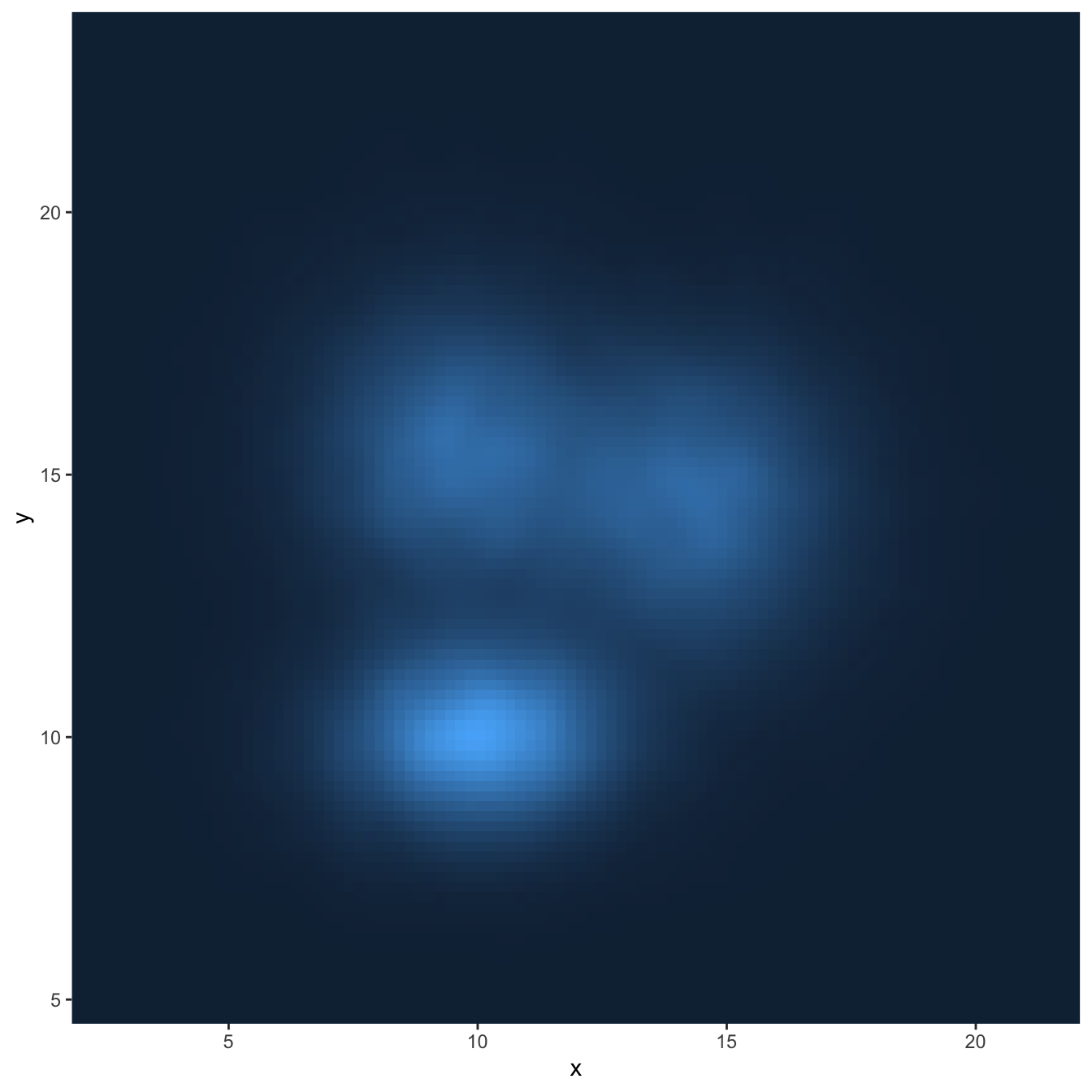As you can plot a density chart instead of a histogram, it is possible to compute a 2d density and represent it. Several possibilities are offered by `ggplot2`: you can show the contour of the distribution, or the area, or use the `raster` function:

``````# Show the contour only
ggplot(data, aes(x=x, y=y) ) +
geom_density_2d()

# Show the area only
ggplot(data, aes(x=x, y=y) ) +
stat_density_2d(aes(fill = ..level..), geom = "polygon")

# Area + contour
ggplot(data, aes(x=x, y=y) ) +
stat_density_2d(aes(fill = ..level..), geom = "polygon", colour="white")

# Using raster
ggplot(data, aes(x=x, y=y) ) +
stat_density_2d(aes(fill = ..density..), geom = "raster", contour = FALSE) +
scale_x_continuous(expand = c(0, 0)) +
scale_y_continuous(expand = c(0, 0)) +
theme(
legend.position='none'
)``````

# Customize the color palette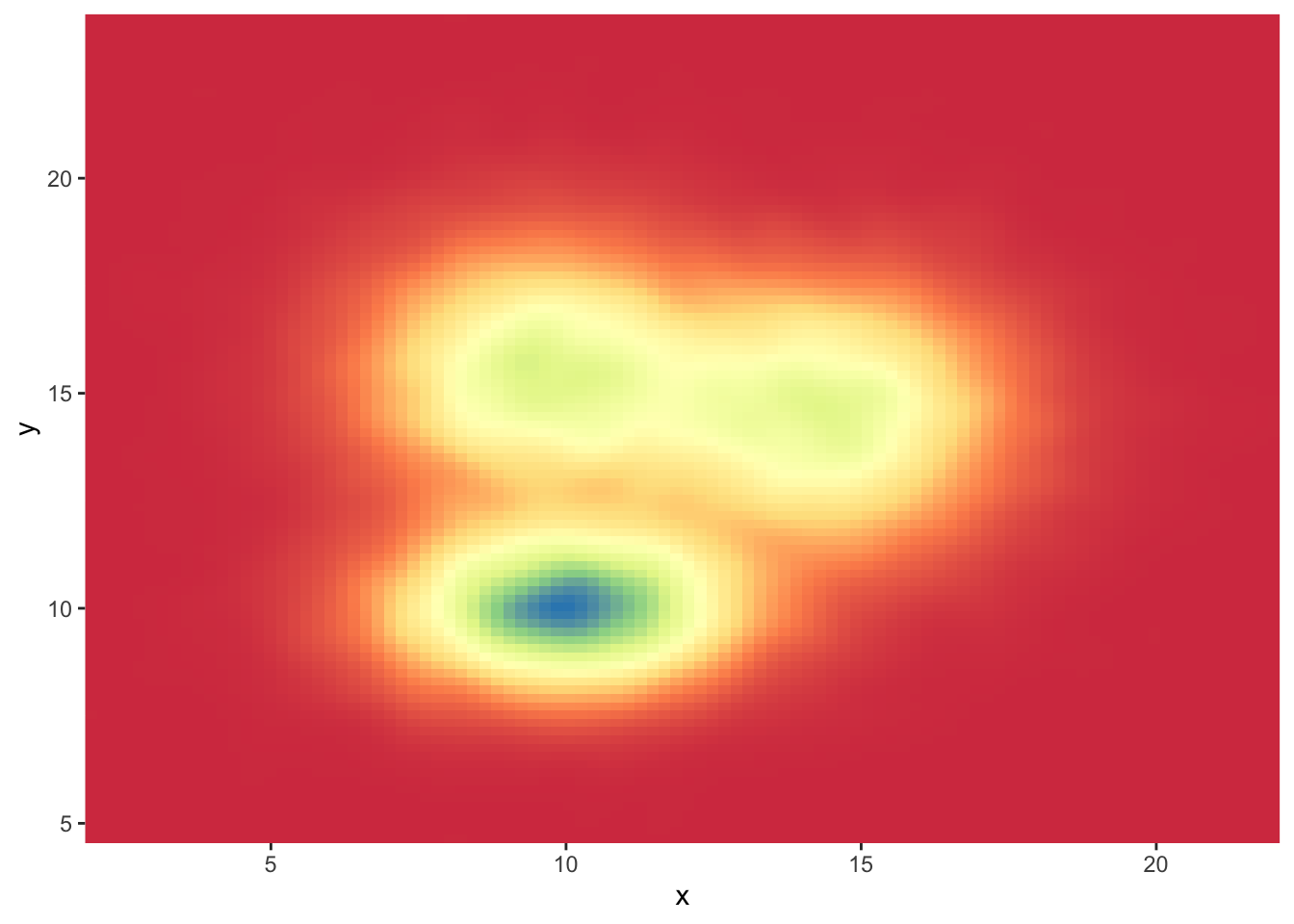Whatever you use a 2d histogram, a hexbin chart or a 2d distribution, you can and should custom the colour of your chart. Here is a suggestion using the `scale_fill_distiller()` function. You can see other methods in the ggplot2 section of the gallery.

``````# Call the palette with a number
ggplot(data, aes(x=x, y=y) ) +
stat_density_2d(aes(fill = ..density..), geom = "raster", contour = FALSE) +
scale_fill_distiller(palette=4, direction=-1) +
scale_x_continuous(expand = c(0, 0)) +
scale_y_continuous(expand = c(0, 0)) +
theme(
legend.position='none'
)

# The direction argument allows to reverse the palette
ggplot(data, aes(x=x, y=y) ) +
stat_density_2d(aes(fill = ..density..), geom = "raster", contour = FALSE) +
scale_fill_distiller(palette=4, direction=1) +
scale_x_continuous(expand = c(0, 0)) +
scale_y_continuous(expand = c(0, 0)) +
theme(
legend.position='none'
)

# You can also call the palette using a name.
ggplot(data, aes(x=x, y=y) ) +
stat_density_2d(aes(fill = ..density..), geom = "raster", contour = FALSE) +
scale_fill_distiller(palette= "Spectral", direction=1) +
scale_x_continuous(expand = c(0, 0)) +
scale_y_continuous(expand = c(0, 0)) +
theme(
legend.position='none'
)``````

Related chart types

## Contact

This document is a work by Yan Holtz. Any feedback is highly encouraged. You can fill an issue on Github, drop me a message on Twitter, or send an email pasting yan.holtz.data with gmail.com.